Published: 30 September 2016

# A novel failure analysis and diagnosis method for hydraulic-turbine generator unit

Xin Xia1
Wei Ni2
1, 2College of Automation, Huaiyin Institute of Technology, Huaian, China
Corresponding Author:
Xin Xia
Views 228

#### Abstract

The failure analysis and diagnosis of hydraulic-turbine generator unit (HGU) is important to protect the safety and stability of the electric system. But failure analysis and diagnosis for HGU is lacking of fault data, and most of the failure analysis and diagnosis methods are proposed without considering the characteristic of HGU. In this paper, a vibration dynamic modeling method for HGU is proposed by using the finite element method, and further the vibration data in different states are getting through numerical simulation. Then, the failure feature is extracted based on the nonlinear output frequency response functions (NOFRFs). Finally, a diagnosis system with Support Vector Machine (SVM) is proposed and employed for diagnosis of the HGU. The experimental results indicate that the feature which extracted from NOFRFs has a strong effect, and demonstrates that the proposed method is feasible and helpful for fault diagnosis in HGU.

## 1. Introduction

The hydraulic-turbine generator unit (HGU) is part of the key equipment in hydropower station. The health state of the HGU is relevant to the safety and stability of the power supply system. Fault in the HGU will not only lead to economic losses but also threaten the safety of people’s life. So, the failure analysis and diagnosis of HGU play an important role in the operation and maintenance which can reduce or eliminate the accident .

According to operating information of the HGU, the failure mainly performs in the form of vibration signals. Researchers have done a lot of work to find the relationship between vibration feature and the failure [1-6]. As hydropower station operating with planned maintenance, some faults are repaired in the early time without record, which makes the vibration data in fault state are difficult to acquire. So there is an urgent need to develop a new method to get faults vibration data of HGU. Some simulation works have been made to get the vibration data. Nelson  have proposed using the finite element method to simulate the dynamic of rotor-bearing system. Shouju Li  have proposed using the finite element method to identify the vibration load. But there is little research on the simulation of faults vibration data of HGU.

Failure analysis and diagnosis methods of HGU can be classified into two categories: signal analysis and modeling methods. Most signal analysis methods are evolved from the diagnosis methods of rotating machinery. Although the HGU belongs to rotating machinery, but it has its own features, such as: low speed, big weight of rotor, variety of types. The signal analysis methods cannot suit each type of HGU very well. In modeling methods, regarding the modeling approach, it is based on a reliable model of the HGU and can be used for the construction of the symptom-fault correlation, or the input-output relation . Recent research has proposed many modeling methods for fault diagnosis [8-12]. Most of them are based on Volterra series and Generalized Frequency Response Function (GFRF). Volterra series is useful in modeling hydroelectric generator unit, but large number of time domain kernels are difficult for fault diagnosis, and the time domain kernels have no physical meaning. GFRF models always encounter the problem of disaster of dimensionality, and high dimensional GFRF have no intuitive feeling to people. LANG [13-15] have proposed non-linear output frequency response functions (NOFRFs) of Volterra systems. This method simplifies the nonlinear frequency domain model, and makes people have an intuitive feeling. However, their work focus on mechanism analysis and few work has done in intelligent diagnosis based on NOFRFs.

Fig. 1The fault diagnostic procedure of HGU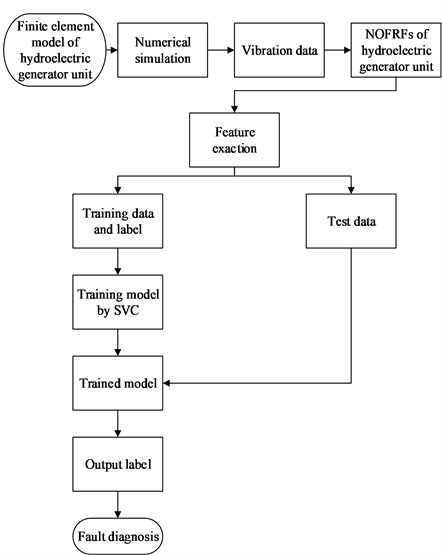In this paper, a novel failure analysis and diagnosis is proposed for HGU. Firstly, in order to overcome the lacking of failure data, a finite element model of a hydroelectric generator unit is proposed, and further the vibration data in different states are acquired based on the model. Secondly, NOFRFs are built for each state, and some quantitative indicators are proposed for NOFRFs as diagnostic features. Finally, a support vector machine (SVM)[16-18] system with binary tree architecture for multi-class classification is built for fault diagnosis. The fault diagnostic procedure is shown in Fig. 1. The proposed method is employed on an experimental study which parameters are totally according to a real HGU. The results indicate that the features which extracted from NOFRFs are much more useful than other diagnosis methods. And the proposed method has a strong effect in failure analysis and diagnosis of HGU.

The rest of paper is organized as follows: in Section 2, the finite element simulation of HGU is presented. In Section 3, the feature extraction and SVM diagnostic system are presented. In Section 4, the proposed method is employed in a HGU which is simplified from a real hydroelectric generator unit, and the proposed is compare to others fault diagnosis methods. Finally, conclusions from this research as well as the advantages and limitations of the proposed method are discussed in Section 5.

## 2. Finite element simulation of vibration response of HGU

### 2.1. Dynamic model of HGU

Failure analysis and diagnosis of HGU plays an important role in the operation and maintenance which can reduce or eliminate the accident. As lacking of fault data, less progress has been made in analysis and diagnostic methods for HGU. With the development of finite element simulation technology, the vibration data of HGU in fault states can be acquired through the numerical simulation.

Fig. 2a) Model of HGU, b) Finite element model of HGU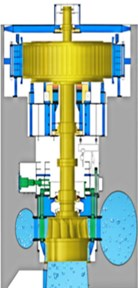a)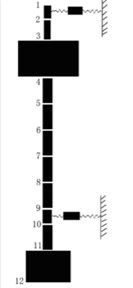b)

Fig. 3The movement of elastic beam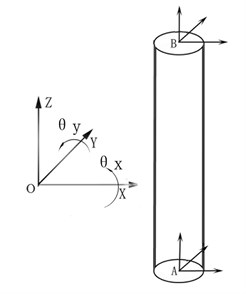Fig. 2(a) shows a HGU, and the structure is quite complex. To simplify the model, the shaft of HGU is set as $N$ elastic beams ($N+1$ nodes, and the node is numbered on the figure) according to the physical structure. The following assumptions are made: the bearing force is linear; the support and foundation are rigid. Then the HGU can be described as Fig. 2(b). In this paper, the parameters of each element have been set according to a real HGU as show in Table 1.

Table 1The parameters of each element after simplified the HGU

 Number 1 2 3 4 5 6 Length (mm) 332 1177 3063.5 1750 1750 1750 Outer diameter (mm) 1170 1170 8664.5 2150 2150 2150 Inner diameter (mm) 730 730 0 1707 1707 1707 Mass (Kg) 1710 6062 980000 18403 18403 18403 Mass per unit length (Kg/mm) 5.15 5.15 319.9 10.516 10.516 10.516 Number 7 8 9 10 11 Length (mm) 1750 1750 750 1750 1462.5 Outer diameter (mm) 2150 2150 2150 2150 6000 Inner diameter (mm) 1707 1707 1707 1707 0 Mass (Kg) 18403 18403 7887 18403 120000 Mass per unit length (Kg/mm) 10.516 10.516 10.516 10.516 82

For each elastic, the moving coordinate system can be set as Fig. 3.

So the movement of each elastic beam can be described as:

1
${X}_{i}=\left[{x}_{Ai}\mathrm{}\mathrm{}\mathrm{}\mathrm{}\mathrm{}\mathrm{}{y}_{Ai}\mathrm{}\mathrm{}\mathrm{}\mathrm{}\mathrm{}{x}_{Ai\theta }\mathrm{}\mathrm{}\mathrm{}\mathrm{}\mathrm{}{y}_{Ai\theta };\mathrm{}\mathrm{}{x}_{Bi}\mathrm{}\mathrm{}\mathrm{}\mathrm{}\mathrm{}\mathrm{}{y}_{Bi}\mathrm{}\mathrm{}\mathrm{}\mathrm{}\mathrm{}{x}_{Bi\theta }\mathrm{}\mathrm{}\mathrm{}\mathrm{}\mathrm{}{y}_{Bi\theta }\right].$

The moving equation of the whole system can be described under usual assumption of time invariant physical properties, and viscous damping as follow [19, 20]:

2
$M\stackrel{¨}{X}+C\stackrel{˙}{X}+KX=F,$

where $M$ is the physical mass symmetric mass matrix which includes translating inertial matrix ${M}_{T}$ and rotating inertial matrix ${M}_{R}$, $C$ is the synthetic damped matrix which includes damping matrix, coupling matrix of support and gyro force matrix. $K$ is the stiffness matrices. $X$ is the vector of displacement responses of elastic beams. $F$ is the external excitation forces.

According to Nelson , The ${M}_{T}$, ${M}_{R}$, $K$ and $C$ are set as follow:

${M}_{T}=\frac{Pl}{420}×\left[\begin{array}{cccccccc}156& 0& 0& 22l& 54& 0& 0& -13l\\ 0& 156& -22l& 0& 0& 54& 13l& 0\\ 0& -22l& 4{l}^{2}& 0& 0& -13l& -3{l}^{2}& 0\\ 22l& 0& 0& 4{l}^{2}& 13l& 0& 0& -3{l}^{2}\\ 54& 0& 0& 13l& 156& 0& 0& -22l\\ 0& 54& -13l& 0& 0& 156& 22l& 0\\ 0& 13l& -13{l}^{2}& 0& 0& 22l& 4{l}^{2}& 0\\ -13l& 0& 0& -3{l}^{2}& -22l& 0& 0& 4{l}^{2}\end{array}\right],$
${M}_{R}=\frac{P{r}^{2}}{120l}×\left[\begin{array}{cccccccc}36& 0& 0& 3l& -36& 0& 0& 3l\\ 0& 36& -3l& 0& 0& -36& -3l& 0\\ 0& -3l& 4{l}^{2}& 0& 0& 3l& -{l}^{2}& 0\\ 3l& 0& 0& 4{l}^{2}& -3l& 0& 0& -{l}^{2}\\ -36& 0& 0& -3l& 36& 0& 0& -3l\\ 0& -36& 3l& 0& 0& 36& 3l& 0\\ 0& -3l& -{l}^{2}& 0& 0& 3l& 4{l}^{2}& 0\\ 3l& 0& 0& -{l}^{2}& -3l& 0& 0& 4{l}^{2}\end{array}\right],$
$K=\frac{EI}{{l}^{3}}×\left[\begin{array}{cccccccc}12& 0& 0& 6l& -12& 0& 0& 6l\\ 0& 12& -6l& 0& 0& -12& -6l& 0\\ 0& -6l& 4{l}^{2}& 0& 0& 6l& 2{l}^{2}& 0\\ 6l& 0& 0& 4{l}^{2}& -6l& 0& 0& 2{l}^{2}\\ -12& 0& 0& -6l& 12& 0& 0& -6l\\ 0& -12& 6l& 0& 0& 12& 6l& 0\\ 0& -6l& 2{l}^{2}& 0& 0& 6l& 4{l}^{2}& 0\\ 6l& 0& 0& 2{l}^{2}& -6l& 0& 0& 4{l}^{2}\end{array}\right],$

where $l$, $r$, $EI$ and $P$ are the length, radius, elasticity modulus and mass per unit length of elastic beam.

$C$ is Rayleigh damping as follow:

3
$C=\alpha M+\beta K,$

where:

4
$\left\{\begin{array}{l}\alpha =\frac{2\left({\xi }_{2}/{\omega }_{n2}-{\xi }_{1}/{\omega }_{n1}\right)}{1/{\omega }_{n2}^{2}-1/{\omega }_{n1}^{2}}\\ \beta =\frac{2\left({\xi }_{2}{\omega }_{n2}-{\xi }_{1}{\omega }_{n1}\right)}{{\omega }_{n2}^{2}-{\omega }_{n1}^{2}}\end{array}\right\,$

where ${\xi }_{1}$ and ${\xi }_{2}$ are damping coefficient that can be taken as 0.1 and 0.2, respectively. And ${\omega }_{n1}$, ${\omega }_{n2}$ are the first and second order critical speed.

It is well know that, some of the nonlinear excitation forces will cause the nonlinear vibration of hydroelectric generator unit. In this paper, we forces on four kinds of typical states of hydroelectric generator unit, as normal state, rubbing state, misalignment state and rubbing-misalignment state. The difference among the models of different states is the external excitation forces. So, the external excitation forces of each states are described as follow:

### 2.1.1. Normal state

In normal state, the excitation force is mainly caused by the initial unbalance mass:

5
$F=Q={M}_{e}{\omega }^{2}r,$

where $Q$ is the initial unbalance mass force, ${M}_{e}$ is initial unbalance mass, $\omega$ is the rotating speed, $r$ is the eccentric radius.

The unbalance mass force is decompose into $x$ and $y$ direction:

6
$\left\{\begin{array}{l}{Q}_{x}={M}_{e}{\omega }^{2}r\mathrm{s}\mathrm{i}\mathrm{n}\left(2\pi \omega \mathrm{}\mathrm{}t+\phi \right),\\ {Q}_{y}={M}_{e}{\omega }^{2}r\mathrm{c}\mathrm{o}\mathrm{s}\left(2\pi \omega \mathrm{}\mathrm{}t+\phi \right),\end{array}\right\$

where $\phi$ is the initial phase.

### 2.1.2. Rubbing state

In rubbing state, because of the discontinuous contacts between rotors and stators, the hydroelectric generator unit present non-linearly phone vibrating phenomena. The excitation forces includes unbalance force and rubbing force:

7
$F=Q+{F}_{r}.$

Han Q.  have proposed the calculation method of partial rubbing force, as follows:

8
$\left\{\begin{array}{l}{F}_{rx}=-{k}_{r}\left(x-{\delta }_{0}\right)h\left(x-{\delta }_{0}\right),\\ {F}_{ry}=-{f}_{r}{k}_{r}\left(x-{\delta }_{0}\right)h\left(x-{\delta }_{0}\right),\end{array}\right\$
9
$h\left(x-{\delta }_{0}\right)=\left\{\begin{array}{l}0,\mathrm{}\mathrm{}\mathrm{}x<{\delta }_{0},\\ 1,\mathrm{}\mathrm{}\mathrm{}x\ge {\delta }_{0},\mathrm{}\end{array}\right\$

where $x$ is radial displacement of rotor, ${\delta }_{0}$ is clearance. For detailed explanations, refer to the research of Han Q. .

### 2.1.3. Misalignment state

In misalignment state, the excitation force includes initial unbalance force and misalignment force:

10
$F=Q+{F}_{m},$

where ${F}_{m}$ is the misalignment force. K. M. Al-Hussain  have explained the misalignment force very detailed. In this paper, we refer to his conclusions.

### 2.1.4. Rubbing-misalignment state

In misalignment state, the excitation force includes unbalance force, misalignment force and rub force, as follow:

11
$F=Q+{F}_{m}+{F}_{r}.$

### 2.2. Numerical simulation

The vibration data of HGU can be get by numerical simulation from Eq. (2). According to the Newmark constant-averaged-acceleration method, Eq. (2) can be discretized into the following scheme :

12
$M{\stackrel{¨}{X}}_{t+\mathrm{\Delta }t}+C{\stackrel{˙}{X}}_{t+\mathrm{\Delta }t}+K{X}_{t+\mathrm{\Delta }t}={F}_{t+\mathrm{\Delta }t},$

where:

${\stackrel{˙}{X}}_{t+\mathrm{\Delta }t}={\stackrel{˙}{X}}_{t}+{\int }_{t}^{t+\mathrm{\Delta }t}{\stackrel{¨}{X}}_{t}tdt,{X}_{t+\mathrm{\Delta }t}={X}_{t}+{\int }_{t}^{t+\mathrm{\Delta }t}{\stackrel{˙}{X}}_{t}tdt,$

where $\mathrm{\Delta }t$ is the step time. Substituting Eq. (12) into Eq. (2), then the derivation of acceleration can be get:

13
$M{\stackrel{¨}{X}}_{t+\mathrm{\Delta }t}=-C{\int }_{t}^{t+\mathrm{\Delta }t}{\stackrel{¨}{X}}_{t}tdt-K{\int }_{t}^{t+\mathrm{\Delta }t}{\stackrel{˙}{X}}_{t}tdt-C{\stackrel{˙}{X}}_{t}-K{X}_{t}+{F}_{t+\mathrm{\Delta }t}.$

The initial conditions are expressed as: ${X}_{t=0}=0$, ${\stackrel{˙}{X}}_{t=0}=0$.

The vibration data can be get by iterative computation through Eq. (2) and Eqs. (12), (13).

The parameters of HGU and the failure parameters are set as Table 2. The initial parameters of numerical simulation are as follows: the rubbing location is at the generator rotor, and the misalignment location is at node 4 (refer to Fig. 2). The initial unbalance mass of HGU locates in runner, and the simulation step is 100 steps per round. The vibration data of node 2 of HGU are collected in different states. The wave and spectrum of simulation vibration are shown in Fig. 4 and Fig. 5.

Table 2Parameters of HGU and the failure parameters

 Parameters of hydroelectric generator unit Failure parameters EI Speed Radius of eccentric mass Rubbing coefficient Angle of misalignment 2.1×1011 N/m2 142.9 rpm 4000 mm 6×109N/m 1o

## 3. Fault diagnosis with NOFRFs-SVM method

### 3.1. Feature extraction based on NOFRFs

HGU belongs to large rotating machinery. The vibration characteristics are similar to many rotor-bearing systems. But it has its own features as well, such as: low speed, big weight of rotor, variety of types. The traditional time-frequency domain analysis methods have difficulty in failure analysis and diagnosis. As seen from Fig. 4 and Fig. 5, the characteristic of different states cannot be described clearly in time-frequency domain. And multi fault is also hard to be explained in the traditional way. So there is an urgent demand for new viewpoints and methods for failure analysis and diagnosis of HGU. In this paper, a novel feature extraction method is proposed based on NOFRFs model for failure analysis and diagnosis.

The vibration model of HGU can be described as a time domain Volterra series, as follow:

14
$y\left(t\right)=\sum _{n=1}^{\mathrm{\infty }}{\int }_{-\mathrm{\infty }}^{\mathrm{\infty }}\cdots {\int }_{-\mathrm{\infty }}^{\mathrm{\infty }}{h}_{n}\left({\tau }_{1},\cdots {\tau }_{n}\right)\prod _{i=1}^{n}u\left(t-{\tau }_{i}\right)d{\tau }_{i},$

where $u\left(t\right)$ is the input vibration data, i.e. the vibration data of lower bearing. $y\left(t\right)$ is the output vibration data, i.e. the vibration data of upper bearing. ${h}_{n}\left(\tau \right)$ is called the $n$th order time domain kernel.

The Eq. (14) is changed with multi-dimensional Fourier Transform, and the frequency domain Volterra series is described as follow:

15
$Y\left(j\omega \right)=\sum _{n=1}^{N}{\int }_{{\omega }_{1}+\cdots +{\omega }_{n}=\omega }{H}_{n}\left(j{\omega }_{1},\cdots j{\omega }_{n}\right)\prod _{i=1}^{n}U\left(j{\omega }_{i}\right)d{\sigma }_{n\omega },$

where $Y\left(j\omega \right)$ and $U\left(j\omega \right)$ are the input and output spectra which are the Fourier Transforms of $u\left(t\right)$ and $y\left(t\right)$. ${H}_{n}$ is called the nth order Generalized Frequency Response Function (GFRF), and can be expressed as Eq. (16):

16
${H}_{n}\left(j{\omega }_{1},\cdots j{\omega }_{n}\right)={\int }_{-\mathrm{\infty }}^{\mathrm{\infty }}\cdots {\int }_{-\mathrm{\infty }}^{\mathrm{\infty }}{h}_{n}\left({\tau }_{1}\cdots {\tau }_{n}\right){e}^{-j\left({\omega }_{1}{\tau }_{1}+\cdots +{\omega }_{n}{\tau }_{n}\right)}\prod _{i=1}^{n}d{\tau }_{i}.$

Fig. 4Time-domain vibration of HGU in each state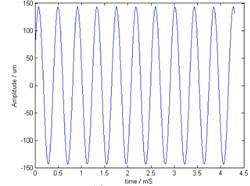a) Normal state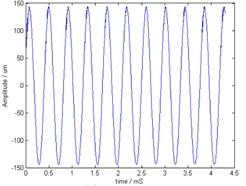b) Rubbing state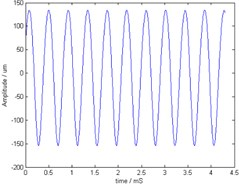c) Misalignment state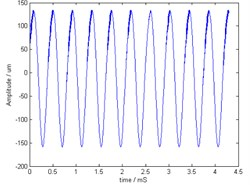d) Rubbing-misalignment state

Fig. 5Frequency domain vibration of HGU in each state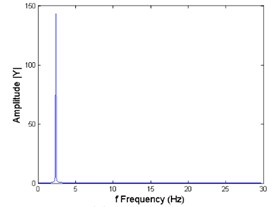a) Normal state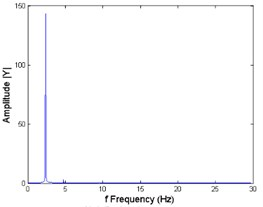b) Rubbing statec) Misalignment state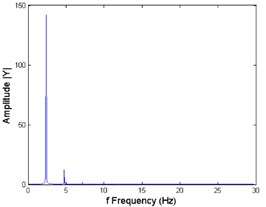d) Rubbing-misalignment state

The $n$th order NOFRFs is given by:

17
${G}_{n}\left(j\omega \right)=\frac{{\int }_{{\omega }_{1}+\cdots +{\omega }_{n}=\omega }{H}_{n}\left(j{\omega }_{1},\cdots ,j{\omega }_{n}\right)\prod _{i=1}^{n}U\left(j{\omega }_{i}\right)d{\sigma }_{n\omega }}{{\int }_{{\omega }_{1}+\cdots +{\omega }_{n}=\omega }\prod _{i=1}^{n}U\left(j{\omega }_{i}\right)d{\sigma }_{n\omega }}.$

Substituting Eq. (16) and Eq. (17) into Eq. (15) lead to:

18
$Y\left(j\omega \right)=\sum _{n=1}^{N}{G}_{n}\left(j\omega \right){U}_{n}\left(j\omega \right).$

When the HGU operates with fault, the change of frequency domain characteristics will react in the NOFRFs. But the NOFRFs always have a wide frequency band, which lead difficult to intelligent fault diagnosis methods. In this paper, we have proposed that use several state characteristics [23, 24] to quantify the feature of NOFRFs.

Frequency center of gravity:

19
$FC=\frac{\underset{0}{\overset{+\mathrm{\infty }}{\int }}j\omega \cdot S\left(j\omega \right)dj\omega }{\underset{0}{\overset{+\mathrm{\infty }}{\int }}S\left(j\omega \right)dj\omega },$

where $j\omega$, $S\left(j\omega \right)$ are frequency and power spectrum of NOFRFs.

Mean square frequency:

20
$MSF=\frac{\underset{0}{\overset{+\mathrm{\infty }}{\int }}\left(j\omega {\right)}^{2}\cdot S\left(j\omega \right)dj\omega }{\underset{0}{\overset{+\mathrm{\infty }}{\int }}S\left(j\omega \right)dj\omega }.$

Frequency domain variance:

21
$VF=\frac{\underset{0}{\overset{+\mathrm{\infty }}{\int }}\left(j\omega -FC{\right)}^{2}\cdot S\left(j\omega \right)dj\omega }{\underset{0}{\overset{+\mathrm{\infty }}{\int }}S\left(j\omega \right)dj\omega }.$

The above state characteristics of each order NOFRFs form the feature vector for failure analysis and diagnosis, as show in Table 3.

Table 3The feature vector based on NOFRFs

 1st order NOFRFs 2st order NOFRFs … Nst order NOFRFs Feature vector FC MSF VF FC MSF VF … FC MSF VF

### 3.2. SVM diagnosis system

Vapnik  introduced Support Vector Machine (SVM). It is a useful method for data classification. It is a learning system based on statistical learning theory which usually separate data into training and testing sets. And in each set, feature data have specific associated with labels. Each of these features of data can be looked upon as a dimension of a hyper-plane. SVM construct a hyper-plane that separates the hyper-space into two classes. SVM algorithm tries to achieve maximum separation between the classes. The classes are separated by a large margin minimizes the expected generalization error.

In this paper, there are four types of state for HGU. Based on the feature vector which proposed in Section 3.1, a SVM with binary tree architecture is built for multi-classification as show in Fig. 6. Firstly, the total samples are classified into two classes, one for the normal state and one for the other states. Secondly, the rest sample is classified into rubbing state and the others. And so on for the rest states. Finally, all four states can be classified by the SVM with binary tree architecture.

Fig. 6SVM diagnosis system with binary tree architecture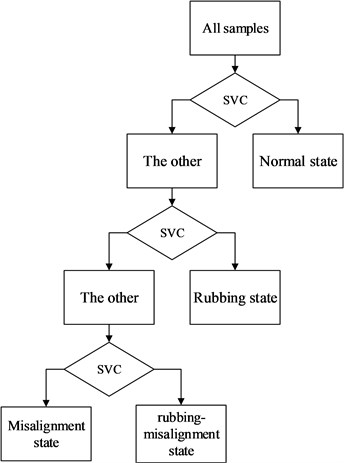## 4. Experimental studies

### 4.1. Verification experiment of the proposed method

The HGU is a complex energy conversion system. According to the energy transfer direction, the vibration of lower guide bearing and upper guide bearing are set as input and output of the mode. In this paper, the vibration data in different states are acquired through finite element simulation which is proposed in Section 2. The vibration data of node 2 is regarded as the vibration data of upper bearing of the HGU, and the vibration data of node 9 is regarded as the vibration data of lower bearing of the HGU. For each of the four states, 20 groups of data are collected, and the sampling length is set as 4096 point/group. The sampling frequency is set as 20 Hz.

Firstly, the traditional method is used to analyze the different fault with the frequency domain spectrum of vibration data of node 2 as showed in Fig. 5. It can be seen that the rubbing state have a very small second harmonic. The misalignment state mainly performance on basic frequency and second harmonic. But for misalignment and misalignment-rubbing state, there are a lot of difficulties to distinguish between them.

To overcome the above problems, the method which is proposed in Section 3 is used for fault diagnosis. For most nonlinear system can be described as 3 order Volterra series , the NOFRFs model is set as 3 order system. The vibration data at node 9 and node 2 are used as input data and output data, respectively. And the NOFRFs models of different states are built. The spectrum of NOFRFs in different states is showed in Fig. 7. It can be seen that normal state mainly performance in first order NOFRFs, the second order and third order NOFRFs has few influence. In rubbing state, the first order NOFRFs focus on basic frequency and multi frequency, the second and third order NOFRFs is promiscuously. In misalignment state, the first and third order NOFRFs focus on the basic frequency, the second order NOFRFs mainly focus on the second harmonic. In misalignment-rubbing state, the rubbing changes the second order NOFRFs compare to misalignment state. So the proposed method has a strong effect to distinguish each state.

### 4.2. Experiment of anti-noise ability of the proposed method

Because of the complicated working condition of the HGU, the measurement data always contain noise jamming in practical engineering. So the verification test of anti-noise ability of the proposed method is necessary.

Fig. 7The NOFRFs of HGU in each state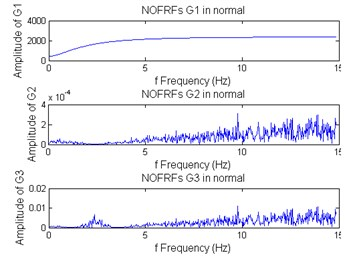a) Normal state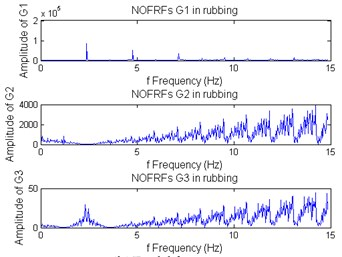b) Rubbing state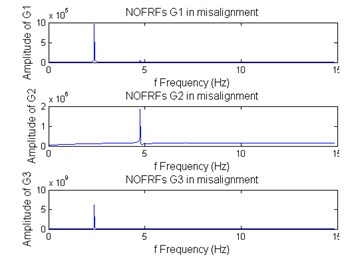c) Misalignment state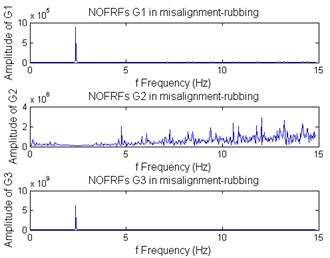d) Rubbing-misalignment state

Fig. 8The NOFRFs of HGU in rubbing-misalignment state with different SNR noise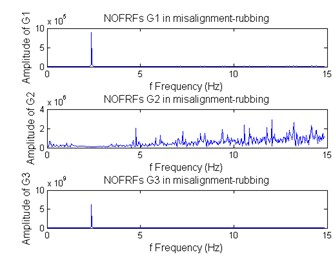a) Without noise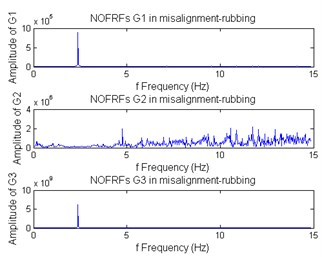b) With noise of 70 dB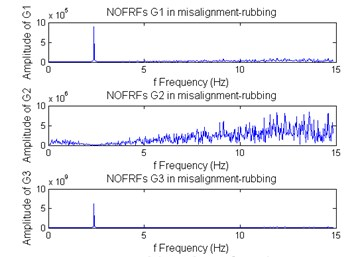c) With noise of 30 dB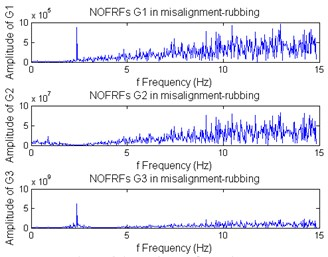d) With noise of 10 dB

In this section, the rubbing-misalignment state is used for the verification. In order to test the anti-noise ability, the white Gaussian noise of different signal-to-noise ratio are added to the measurement input-output data. The signal-to-noise ratio is set as 70 dB, 30 dB, 10 dB. The spectrum of NOFRFs in different SNR is showed in Fig. 8. It can be seen that the system’s character is performed clear even there exists noise interference.

The result indicates that the proposed method has a strong ability of restraining noise. Further pointed out that the proposed method is suitable for engineering practice.

### 4.3. Comparison experiment

In this section, the proposed method is compared to the wavelet analysis method and frequency domain spectrum analysis method to demonstrate the effectiveness of this method. For the proposed method, the feature is extracted with the method which proposed in Section 3.1. The 2-dimension distribution of NOFRFs features is shown in Fig. 9. And for the wavelet analysis method and frequency domain spectrum analysis method, the feature is extracted from the wavelet coefficient and frequency domain respectively.

Fig. 9The 2-dimension distribution of NOFRFs features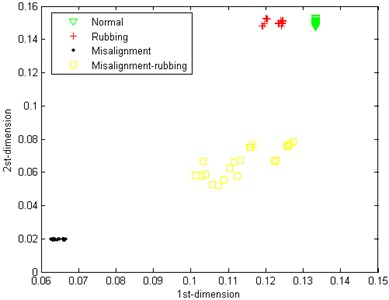In order to achieve the intelligent fault diagnosis and compare the proposed method to other fault diagnosis method, the SVM fault diagnosis system is proposed to verify the accuracy rate of the different methods. For the 20 groups of collected vibration data, 20 groups of feature are extracted for each method. Five groups of NOFRFs feature and traditional feature of each state are used as training data for the SVM fault diagnosis system respectively. And all groups of data of each state are used to test the fault diagnosis system. The results are showed in Table 4.

Table 4Fault diagnosis result of SVM system by different diagnosis methods

 The proposed method The wavelet analysis method The frequency domain spectrum analysis method First SVM 0 0 1 Second SVM 0 1 0 Third SVM 0 1 6 Accuracy rate 100 % 97.5 % 91.25 %

The results indicate that the proposed method is useful for fault diagnosis in HGU.

## 5. Conclusion

Failure vibration data of HGU are difficult to acquire. And the traditional failure analysis and diagnosis methods ignore the characteristic of HGU which makes the failure analysis and diagnosis less effective. To overcome these problems, finite element method is used to simulate the vibration data of the HGU unit in different states, and a novel failure analysis and diagnosis method is proposed for HGU. The research work is conducted in three phases. Firstly, a finite element model is proposed according to the physical parameters of a real HGU, and the vibration data are acquired through numerical simulation. Secondly, a novel feature extracted method is proposed, and the feature in different state is extracted. Thirdly, a SVM system with binary tree architecture is built for fault classification. Finally, the proposed method is successfully employed on an experimental study. The results indicate that the proposed method can describe the failure characteristic better than the traditional time-frequency analysis method, and it is suitable for fault diagnosis of HGU.

Future research is to improve the computational efficiency and apply this method to practical engineering diagnostic cases.

#### Cited by

A new method for axis adjustment of the hydro-generator unit using machine learning
Condition-based maintenance in hydroelectric plants: A systematic literature review
Sensitivity analysis of a Pelton hydropower station based on a novel approach of turbine torque

06 January 2016
Accepted
28 June 2016
Published
30 September 2016
SUBJECTS
Fault diagnosis based on vibration signal analysis
Keywords
hydraulic-turbine generator unit
fault diagnosis
finite element
nonlinear output frequency response functions
Acknowledgements

This work is supported by National Science Foundation for Distinguished Young Scholars of China (61203056). Platform Construction Project of Technology Innovation of Huaian China (HAP201432). We greatly thank our university for their great assistance to our study and helpful suggestions to the presentation of this paper.